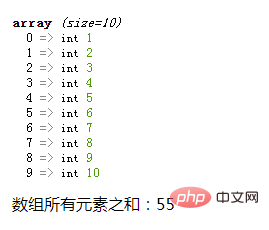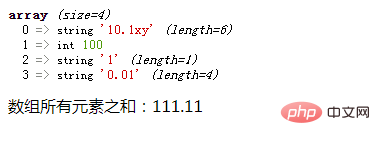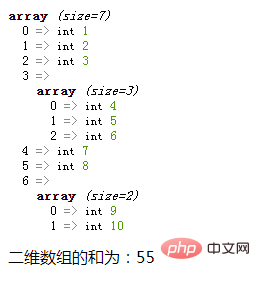# php数组求和的函数是什么

php数组求和的函数是“array_sum()”；该函数可以计算一维数组中所有元素的和，并返回元素之和，语法“array_sum(\$array)”。如果“\$array”中所有元素都是整数，则返回一个整数值；如果其中有一个或多个值是浮点数，则返回浮点数。如果“\$array”中存在非数值类型的元素，那么PHP会将它们转换成一个数值，转换失败就作为0值来参加计算。php入门到就业线上直播课：进入学习

php数组求和的函数是“array_sum()”。

php数组 array_sum()函数

array_sum()函数可以计算数组中所有元素的和，并返回元素之和。

```<?php
\$array= array(1,2,3,4,5,6,7,8,9,10);
var_dump(\$array);
echo '数组所有元素之和：'. array_sum(\$array);
?>```• 如果`\$array`中所有元素都是整数，则返回一个整数值；如果其中有一个或多个值是浮点数，则返回浮点数。

• 如果`\$array`中存在非数值类型的元素，那么PHP会将它们转换成一个数值（PHP是弱语言类型，会根据变量的值，自动把变量转换为正确的数据类型），转换失败就作为 `0` 值来参加计算。

```<?php
\$array= array("10.1xy", 100, '1', "0.01");
var_dump(\$array);
echo '数组所有元素之和：'. array_sum(\$array);
?>```• 使用foreach语句循环遍历二维数组的外层数组元素

• 在循环体，使用is_array()函数判断外层元素是否为数组类型，并利用“+=”运算符求和

```<?php
\$arr = array(1,2,3,array(4,5,6),7,8,array(9,10));
var_dump(\$arr);
\$sum=0;
foreach(\$arr as \$v){
if(is_array(\$v)){
\$sum+=array_sum(\$v);
}else{
\$sum+=\$v;
}
}
echo "二维数组的和为：".\$sum;
?>```1/1